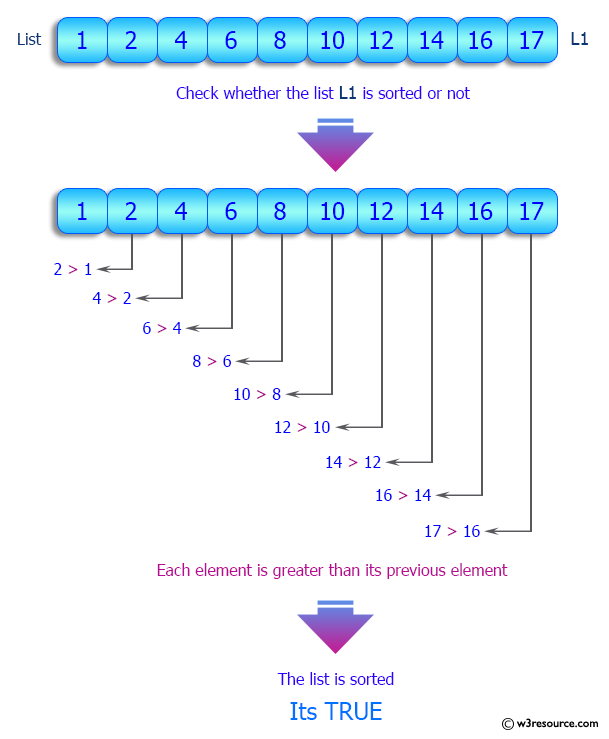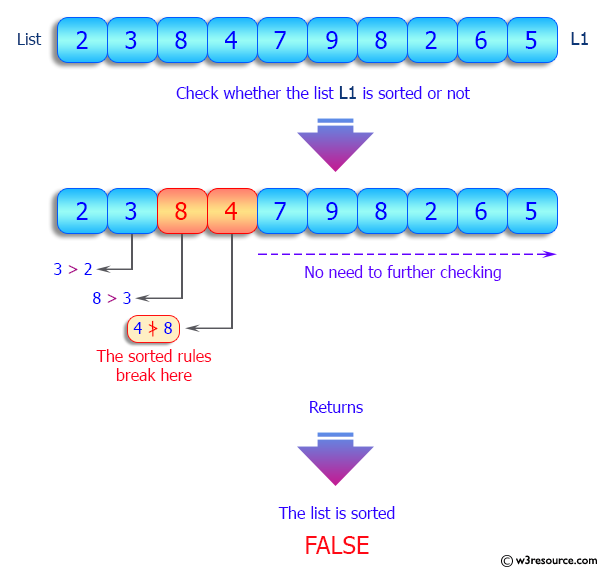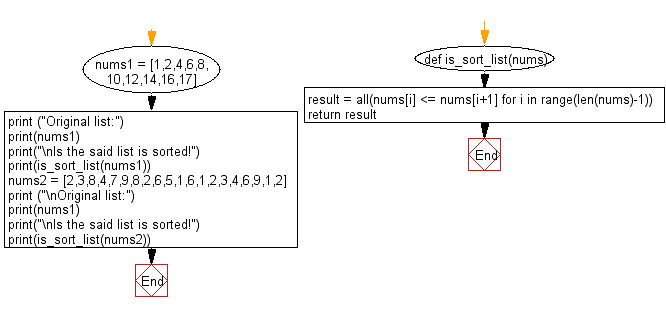﻿ Python: Check whether a specified list is sorted or not - w3resource# Python: Check whether a specified list is sorted or not

## Python List: Exercise - 112 with Solution

Write a Python program to check whether a specified list is sorted or not.

Sample Solution:

Python Code:

``````def is_sort_list(nums):
result = all(nums[i] <= nums[i+1] for i in range(len(nums)-1))
return result

nums1 = [1,2,4,6,8,10,12,14,16,17]
print ("Original list:")
print(nums1)
print("\nIs the said list is sorted!")
print(is_sort_list(nums1))

nums2 = [2,3,8,4,7,9,8,2,6,5,1,6,1,2,3,4,6,9,1,2]
print ("\nOriginal list:")
print(nums1)
print("\nIs the said list is sorted!")
print(is_sort_list(nums2))
```
```

Sample Output:

```Original list:
[1, 2, 4, 6, 8, 10, 12, 14, 16, 17]

Is the said list is sorted!
True

Original list:
[1, 2, 4, 6, 8, 10, 12, 14, 16, 17]

Is the said list is sorted!
False
```

Pictorial Presentation:Flowchart:## Visualize Python code execution:

The following tool visualize what the computer is doing step-by-step as it executes the said program:

Python Code Editor:

Have another way to solve this solution? Contribute your code (and comments) through Disqus.

What is the difficulty level of this exercise?

Test your Programming skills with w3resource's quiz.

﻿

## Python: Tips of the Day

Invokes the provided function after ms milliseconds:

Example:

```from time import sleep

def tips_delay(fn, ms, *args):
sleep(ms / 1000)
return fn(*args)
print(tips_delay(
lambda x: print(x),
1000,
'w3r'
))
```

Output:

```w3r
None
```